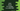# Java program to check if a number is valid IMEI or not## Java program to check if a number is valid IMEI or not:

IMEI number is an unique number for each mobile phones. You can start your dial-pad and enter *#06# to check the IMEI on your phone. It is an unique number and only phone manufacture companies have the authority to register it with a phone.

The IMEI number is 15 digits number. 14 digits plus one check digit. The check digit is calculated by using an algorithm, known as Luhn algorithm. This digit is calculated by doing some calculations on the remaining digits.

There is a way to validate the check digit is a valid digit or not. If the check digit is valid, we can say that the IMEI is valid. Else it is not.

In this post, we will write one Java program that will take one IMEI number as an user-input and validate if it is a valid IMEI or not.

### Algorithm to check if a number is valid IMEI:

We need to do a simple calculation to check if a number is a valid IMEI. The below algorithm we need to follow:

• Starting from the rightmost digit, double every alternate digit.
• If we get a number in two digits after doubling the digit, add the digits of the number to get a one digit value.
• Once the final digit is found, check if it is divisible by 10 or not. Or, if we divide the number by 10, if it give 0, it will be a valid IMEI.

### Example of IMEI validity check:

Let’s take a look at the example IMEI 490154203237518.

Pick the digits from right to left and double all alternate digits:

``````8 = 8
1 = 1*2 = 2
5 = 5
7 = 14 = 1 + 4 = 5
3 = 3
2 = 4
3 = 3
0 = 0
2 = 2
4 = 8
5 = 5
1 = 2
0 = 0
9 = 18 = 9
4 = 4``````

The sum is: 8 + 2 + 5 + 5 + 3 + 4 + 3 + 0 + 2 + 8 + 5 + 2 + 0 + 9 + 4 = 60. Since 60 is divisible by 10, it is a valid IMEI.

### Java program:

Below is the complete Java program that takes the IMEI value as input and prints if it is valid or not:

``````import java.util.Scanner;

class Main {

public static int getSumDigits(int n) {
int sum = 0;
while (n > 0) {
sum += n % 10;
n = n / 10;
}
return sum;
}

public static boolean isValidImei(long imei) {
int imeiLength = Long.toString(imei).length();

if (imeiLength == 15) {
int sum = 0, lastDigit;
for (int i = imeiLength - 1; i >= 0; i--) {
lastDigit = (int) (imei % 10);

if (i % 2 == 1) {
lastDigit = getSumDigits(2 * lastDigit);
}
sum += lastDigit;
imei = imei / 10;
}

return sum % 10 == 0;
}

return false;
}

public static void main(String[] args) {
long imei;
Scanner sc = new Scanner(System.in);

System.out.println("Enter the IMEI value: ");
imei = sc.nextLong();

if (isValidImei(imei)) {
System.out.println("Valid IMEI");
} else {
System.out.println("Invalid IMEI");
}
}
}``````

### Explanation:

Here,

• getSumDigits method is used to find the sum of digits of a number.
• isValidImei method is used to check if an IMEI value is valid or not. It takes the IMEI value as its argument and returns one boolean value. true for valid and false for invalid.

• It checks if the length is 15 or not. If it is not 15, it returns false.
• By using a for loop, it finds the sum of digits of the number. It uses the same algorithm we have discussed above.
• Once the sum is calculated, it checks if the sum is divisible by 10 or not. If yes, it returns true. Else, it returns false.
• Based on the return value of isValidImei, we are printing a message to the user.

### Sample output:

If you run the above program, it will give output as like below:

``````Enter the IMEI value:
490154203237518
Valid IMEI

Enter the IMEI value:
1234567890989
Invalid IMEI``````